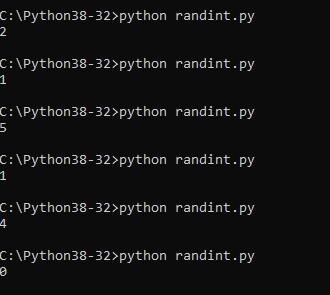Home » The Random Module in Python

# The Random Module in Python

The ability to generate random numbers or strings is very important in programming languages whether its a game where you generate enemies in a random position, a password generator where you obviously would like to generate random passwords or maybe even a lottery number generator.

In this article we look at how you can generate random numbers and strings (among other things) using python. Lets get started

To achieve this we look at the random module and some its very useful functions, lets look at the first one randint

### randint example

randint accepts two parameters: a lowest and a highest number. It returns a random integer N such that a <= N <= b.

Lets look at an example

```#!/usr/bin/python
import random
​print (random.randint(0, 5))```

If you run this you should see something like the following, I ran this a few timesrandint output

### randrange example

randrange() returns a randomly selected element from range(start, stop, step)

start − Start point of the range. This is included in the range.
stop − Stop point of the range. This is excluded from the range.
step − Steps to be added in a number.

Lets look at an example

```#!/usr/bin/python
import random

# Select an even number in 1 <= number < 100
print ("randrange(1, 100) : ", random.randrange(1, 100))

# Select an even number in 1 <= number < 100
print ("randrange(1, 100, 2) : ", random.randrange(1, 100, 2))

# Select a number in 1 <= number < 100
print ("randrange(1, 100, 3) : ", random.randrange(1, 100, 3))```

If you run this you should see something like the following, I ran this twicerandrange

### Choice example

You can use choice to get a random item from a list, tuple, or string. Lets take a look at an example

```#!/usr/bin/python
import random

print ("choice([1, 2, 3, 4, 5]) : ", random.choice([1, 2, 3, 4, 5]))
print ("choice('abcdefghijklmnopqrstuvwxyz') : ", random.choice('abcdefghijklmnopqrstuvwxyz'))```

If you run this you should see something like the following, I ran this three timeschoice output

0 comment

#### You may also like

This website uses cookies to improve your experience. We'll assume you're ok with this, but you can opt-out if you wish. Accept Read More